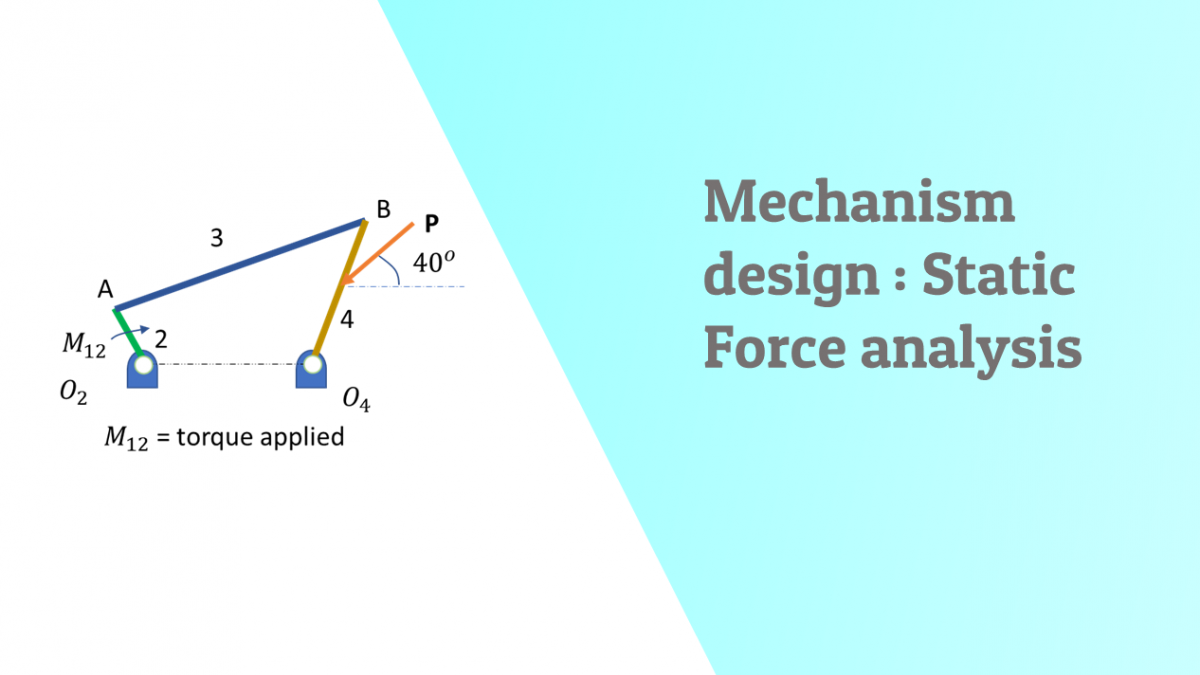# Static Force analysis on a 4 bar link mechanismHow to solve a static force analysis problem for a typical 4 bar link mechanism in static condition

Static force analysis for a 4 bar link mechanism at rest .

Parameters known :

Lengths and orientation of all the links ,

Force P in magnitude and direction.

Link 1 is fixed, Link 2 is the crank with a torque M applied

Force P is a force acting on Link 4 which holds the 4 bar mechanism in static equilibrium .

How to calculate the Moment M if applied force P is known to maintain equilirbrium of the system ?

Step 1 :

Free body diagrams of the links

Step 2 :

Find force F34 on Link 4

Moment balance equation for Link 4 :

x, y are known through geometry  and P is input,  F34 can be found.

Step 3 :

Find the reaction at Link 4

With known F34 in magnitude and direction and also known P in magnitude and direction. Find the intersection point. Connect intersection point to point B . Connect point O4 to the intersection point. That is direction of F14.

Complete Force triangle for 3 force member.

Find the unknown force F14 in magnitude and direction.

Step 4 : Finding the Moment

On Free body diagram of Link 3

F34 = -F43 =F23

On Link 2 , F32 = -F23

When F32 is known, we can find Moment M and reaction F12 .

Reaction F12 = -F32 equal and opposite as there are no other forces on link 2.

Moment balance equation for Link 2 .

z is perpendicular distance to F32 from O2. Known from geometry.

Since F32 is also known the Moment can be calculated.

This gives the Torque required to be applied at the crank for static equilibrium.

Categories: Mechanism design